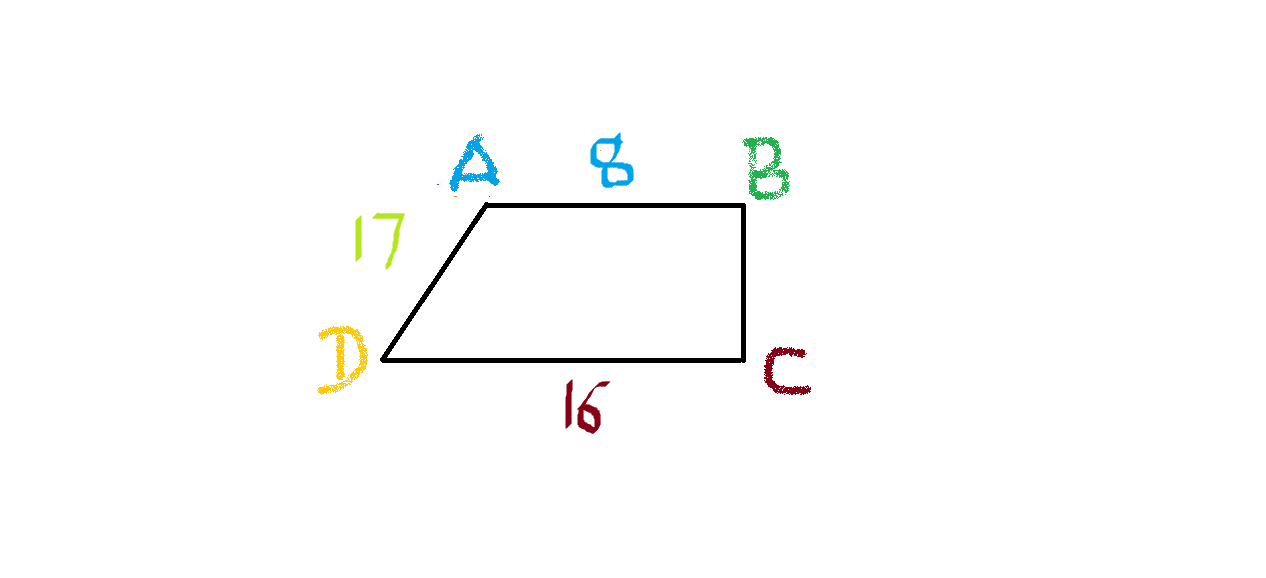# Can you find BC?

Geometry Level 2In a given figure, $ABCD$ is a trapezium in which the parallel sides $AB$ and $DC$are both Perpedicular to $BC$. If $AB=8$, $AD=17$ and $CD=16$, then $BC$ is

×